The model “AtmoSym» of atmospheric processes is based on the following complete set of three-dimensional non-stationary hydrodynamic equations for an atmospheric gas: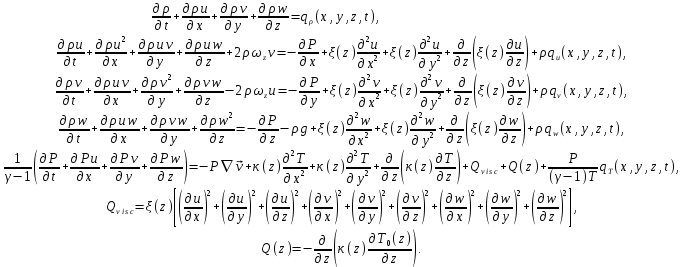(1)

In the model (1),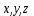are horizontal and vertical coordinates;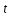is time,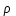is density;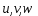are horizontal and vertical components of velocity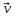;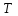is temperature;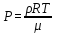is pressure;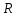is the universal gas constant;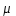is a molecular weight;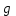is the acceleration of gravity,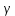is an adiabatic index;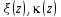are coefficients of viscosity and thermal conductivity;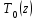is a vertical profile of the background temperature;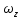is a local vertical component of the angular velocity of gyration of the Earth.

The equations take into account non-linear and dissipative processes accompanying wave propagation; they, in particular, describe such complicated phenomena as formation of shock waves, wave breaking, turbulence.

The model ensures self-consistent description of atmospheric processes and takes into account change in time of atmosphere parameters due to energy transfer from dissipated waves to the medium.

## Background state of the atmosphere and used empirical model

The background profilesand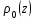are taken from the empirical model NRLMSIE-00. Each user has to enter some data-ins of empirical model NRLMSIE-00 being if interest for him. .

In the last equation, the term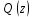is a complementary heat source ensuring stationarity of the empirical nonisothermal atmosphere in conditions of lack of wave disturbances. (That is, when perturbations are absent, the state of the atmosphere corresponding to the NRLMSIE-00 empirical model is being maintained.)

## Boundary conditions

### Conditions on the upper bound

On the upper bound (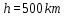) of the simulated field of the atmosphere, the boundary conditions being standard for the problems of propagation of wave disturbances in the thermosphere are imposed: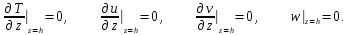(2)

These boundary conditions are explained traditionally by large viscosity and heat conductivity at the altitudes of order of 500 km and above.

### Conditions on the lower bound

The equations (and the program) permit two various versions of the lower boundary conditions. In both optional versions of boundary conditions, because of the viscosity is taken into account, one has to impose some conditions on a horizontal velocity of an atmospheric gas on the lower boundary. In many cases, the following conditions are reasonable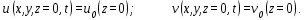(3)

Here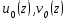are vertical profiles of a background wind.

Owing to a small role of viscosity near the Earth’s surface, the boundary conditions (3) have essential effect only within of some thin layer near the Earth’s surface. Estimates show that possible reasonable modifications of the boundary conditions (3) have weak influence on the processes in the whole atmosphere, except for a thin layer near the Earth’s surface. Therefore, improving the conditions (3) is senselessly.

Now we consider the boundary conditions, which have great influence on the atmospheric gas behavior. There are two possible essentially various types of the lower boundary conditions.

The lower boundary condition of the first type

As one of the lower boundary conditions, one can impose the condition on a vertical gas velocity on the lower bound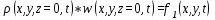(4)

where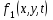is a function given on the lower bound.

The impenetrability condition of the Earth’s surface is the most frequent condition of such a type; in this case the condition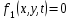is applied.

If waves propagate from some source on the Earth’s surface, (for example, if we consider waves from earthquakes) then obviously the case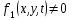is of interest.

Together with the condition (4), some condition on temperature is necessary to set. For example, often the condition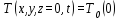(5)

is comprehensible

It is important to note that, owing to a small role of thermal conductivity near the Earth surface, the last condition influences the gas behavior only within a thin layer near the Earth’s surface. Therefore, any improvement of the condition (5) is senseless.

The lower boundary condition of the second type

In the second case, on the lower boundary, the following conditions are given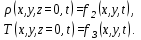(6)

However, one can show that only the pressure variations on the lower bound essentially determine the solution behavior in the whole atmosphere.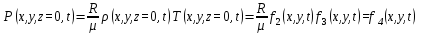, (7)

while the concrete conditions (6) on temperature and density influence on the solution behavior only in a thin layer near the Earth’s surface. Consequently, any details of conditions (6) on density and temperature on the lower boundary are not relevant.

### Boundary conditions on the left and right bounds

In the model, periodic boundary conditions on the left and right bounds are sewn up. We have chosen periodic conditions due to the following reasons:

Such conditions are simple and serviceable

If we consider waves from a local source, then the bounds always can be taken moved away in order that they have not influence on the considered solution

Periodic conditions are suitable for the problems of vertical propagation of waves

These periodic conditions are as follows. Let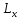be a size of considered region along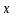-axis, and let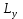be a size of considered region along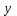-axis. Then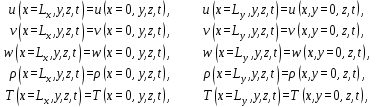## Initial conditions

The model needs five initial conditions: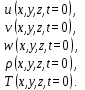In the elementary version, it may be the conditions of lack of waves at an initial instant: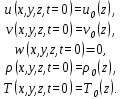## Wave sources

The possibility of assignment of wave sources is provided in the model. Each user can define any of the wave sources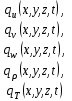in case, if the considered problem requires introducing of some wave sources. Within an elementary version of the model, all these functions are equal zero.

Consideration of the source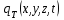can allow, for example, taking into account the heating/cooling of an atmospheric gas at the expense of energy input at changes of the water phases.

## Wind

The model allows consideration of the wind effects on atmospheric processes. The user can set a vertical wind-profile in the program as follows. In the initial conditions of the model, the user takes that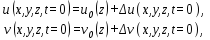whereare functions describing a vertical wind-profile, and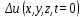, are the functions describing wave components of horizontal velocities at t=0.

In the elementary version, the user can take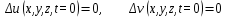## Remarks

The described model has a two-dimensional version which can be simply called. The two-dimensional version of the model is economic.

The model is under continuous perfection. Therefore, the real computer model can be more complicated than the one described here. For example, we suppose to add in the near future some special block of subprograms to consider transport equations for water in different phases. We plan to include an equation for a water vapor, an equation for finely divided water, and an equation for ice particles. Correspondingly, we plan to take into account heating/cooling of an atmospheric gas at the expense of energy input at changes of water phases. Such a block will allow studying some meteorological processes (for example, formation of clouds, also convection and tornado) and their influence on the upper atmosphere. We suppose also to consider the influence of the ionosphere on the processes in a neutral component of the atmosphere.

The use and control of the model is carried out with some press-button system. It allows cutting off easily those processes that are uninteresting to the user. Therefore, any perfections of the model do not bind any user to use difficult versions of the model, but simply they do the model to be universal.

Main Page